CYBER MONDAY! 25% storewide! (\$5 min.)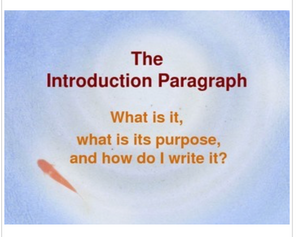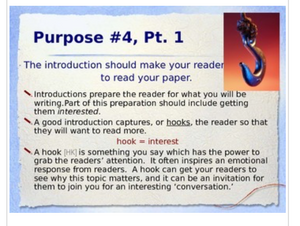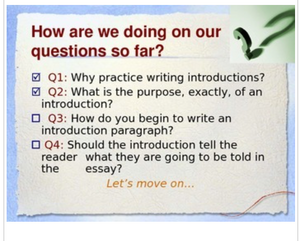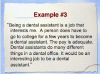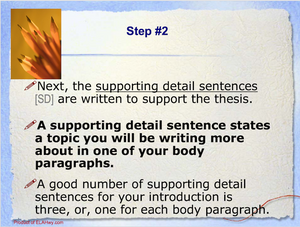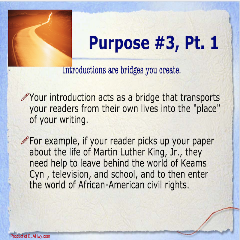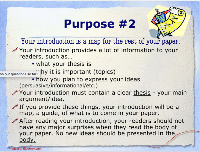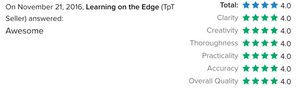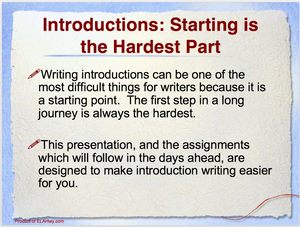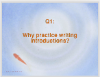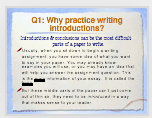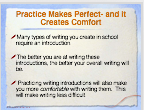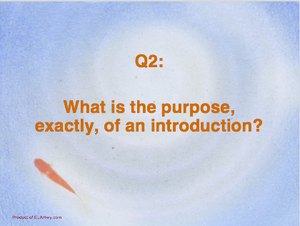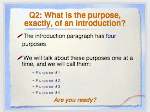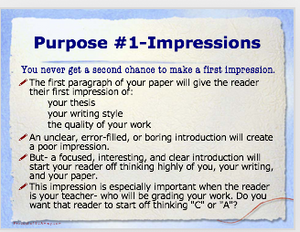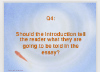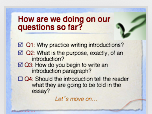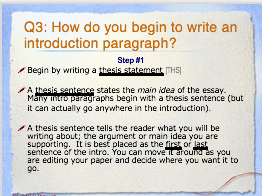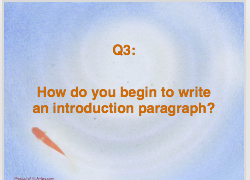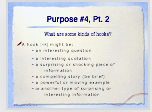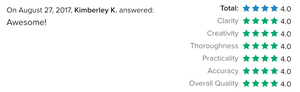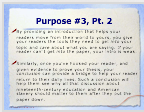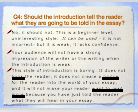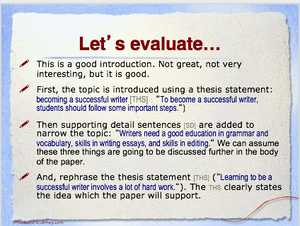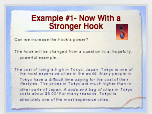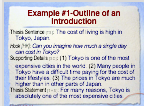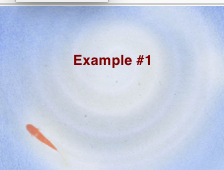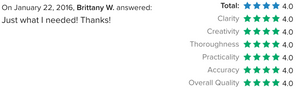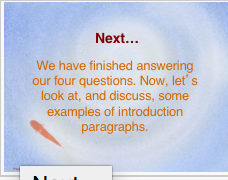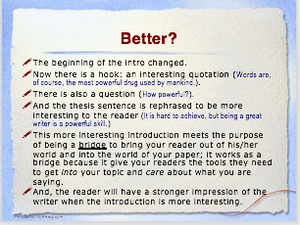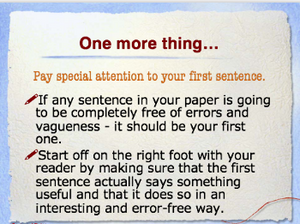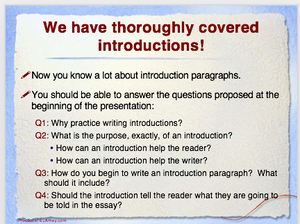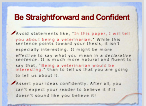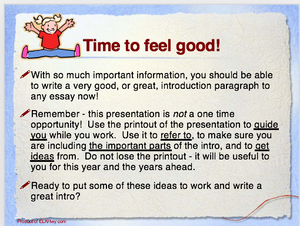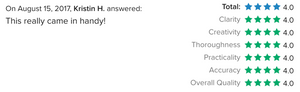# Introduction Paragraphs: PowerPoint Presentation

Regular price \$14.00 Sale

A must for secondary ELA teachers!
43 slides, sound and motion effects throughout

Walks students through the following central ideas, then goes into detail about each:
> Purposes of an introduction
> Parts of an introduction (thesis, supporting topics, hook, restatement of thesis)
> Hooks (what are they, examples of)
> Among others, it answers the following essential questions:
•     Why should you practice writing introductions?
•     What is the purpose of an introduction?
•     How can an introduction help the reader?
•     How can an introduction help the writer?
•     How do you begin to write an introduction paragraph? What should it include?
> Includes many of example intros with step by step improvements. These examples are designed to be interactive, with students answering questions on the slides as you go.
> Includes metaphors with loads of details and examples to reinforce value of intro ("Your introduction is a map for the rest of your paper.").